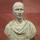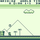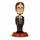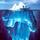## General Question# Can you please help me out with this maths question?

Asked by FireMadeFlesh (16548) September 8th, 2010

Yes, this is a homework question. The thing is, I am the tutor, and it is my student’s question.

If y = 0.5(a^b + a^-b), show by substitution that loga(y + sqrt(y^2 – 1)) = b.

loga is log base a, and sqrt is the square root of y^2 – 1.

I’m not quite sure what they mean by “by substitution”, or exactly which direction I should take this in. Thanks in advance!

Observing members: 0Composing members: 0By substitution means they moved everything but the value of b to the left side, since the formula is no longer the same, but the values of the variables remain the same so it doesn’t matter. They want you to prove the second formula is correct in the process of conversion.

Also you should resign for being incompetent. I’m not trying to insult you, but your student is probably better off with someone who already knows this stuff.

Zyx (4155)“Great Answer” (0) Flag as…@Zyx That is not substitution, that is just rearranging. “By substitution” implies that there is another term or expression I can use to make the question more manageable. I’m also not incompetent, but am a little short on time to prepare for lessons. He also has my textbook to study from, so I can’t look it up. I have been teaching this student for a while, and this is the first question to give me trouble.

FireMadeFlesh (16548)“Great Answer” (3) Flag as…I haven’t used logarithm’s for a “very” long time but my assumption is that you would have to change your log base a equation to a^b = (y + sqrt(y^2–1)). Substitute your y() equation into this and solve. Not sure if you’ll be able to reduce enough to prove. Probably have to use some theories I’ve totally forgotten but that may help get you started.

@Zyx: Really?? Your own incompetence precedes you, and has been proven by your comment above.

critter1982 (4120)“Great Answer” (1) Flag as…[loga(y + sqrt(y^2 – 1) = b] also equals [a^b = (y + sqrt(y^2–1))].
So now your 2 equations are a^b = (y + sqrt(y^2–1)) and y = 0.5(a^b + a^-b).

Now y = 0.5(a^b + a^-b) also equals y = 0.5(a^b + (1/a^b).

So now your 2 equations are a^b = (y + sqrt(y^2–1)) and y = 0.5(a^b + (1/a^b)).

Substituting in the 2nd equation yields: y = 0.5[(y + sqrt(y^2–1)) + (1/(y + sqrt(y^2–1)))].

Now multiply both sides by sqrt(y + y^2 -1) this yields:
y(y + sqrt(y^2–1)) = 0.5 [ y^2 + y(sqrt(y^2–1)) + y(sqrt(y^2–1)) + y^2 -1 + 1]

reducing both sides:

y^2 + y(sqrt(y^2–1)) = 0.5y^2 + 0.5*y(sqrt(y^2–1)) + 0.5y(sqrt(y^2–1)) + 0.5y^2

still reducing:

y^2 + y(sqrt(y^2–1)) = y^2 + y(sqrt(y^2–1))

Both sides are the same which proves these equations to be true.

NEXT…..

critter1982 (4120)“Great Answer” (2) Flag as…@critter1982 I’d hardly consider giving bad advice online incompetence xD
Also it might be worth mentioning I’m about to attempt high school math for the third time xD

Zyx (4155)“Great Answer” (0) Flag as…My point exactly…you were unqualified to answer a question in which you answered anyways (incorrectly)...and of which had nothing really to do with the question anyways.

critter1982 (4120)“Great Answer” (0) Flag as…given:
y = ( a^b + a^-b ) / 2

y^2 = (¼)( a^2b + 2 + a^-2b)
y^2 – 1 = y^2 – 4/4 = (¼)(a^2b – 2 + a^2b)
which is still a perfect square. Therefore
y^2 – 1 = (a^b – a^-b)^2 / 4
so taking the square root is easy.
sqrt ( y^2 – 1 ) = (a^b – a^-b) / 2

Thus
y + sqrt ( y^2 – 1) = (½)( a^b + a^b + a^-b + a^-b – a^-b)
y + sqrt ( y^2 – 1) = (½)( 2a^b)
y + sqrt ( y^2 – 1) = a^b

Now take log base a (call it loga) of both sides and you’re done.
loga( y + sqrt(y^2 – 1)) = loga( a^b)
loga( y + sqrt(y^2 – 1)) = b

gasman (11296)“Great Answer” (2) Flag as…Btw, the quantities ( e^x + e^-x ) / 2 and ( e^x – e^-x ) / 2—which has the form of y in this problem—are the formulas for hyperbolic cosine and sine functions, respectively. Using imaginary exponents they can stand in for ordinary trigonometric cosine & sine as well.

gasman (11296)“Great Answer” (2) Flag as…@critter1982 and @gasman Thanks so much, that is a great help.

FireMadeFlesh (16548)“Great Answer” (0) Flag as…or